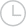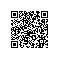使用XL翼将1列从100个不同的Excel路径复制到1个Excel文件一码平川MACHEL2019-01-22 16:37:15366

wb=xw.Book()
for i in ripPaths:

wbtemp=xw.Book(i)
my_values=wbtemp.sheets['sheet1'].range('B5:B4722').options(ndim=2).value
wb.sheets['sheet1'].range("A1:A4722").value=my_values取消 提交回答

• 一码平川MACHEL
2019-07-17 23:26:19

当然，这是我的看法。这类事物的信件很复杂，因为Excel可以从AZ循环，然后是AA-AZ等，但是：这是一个方法：

wb=xw.Book()
for i in range(len(ripPaths)):

wbtemp=xw.Book(ripPaths[i])
my_values=wbtemp.sheets['sheet1'].range('B5:B4722').options(ndim=2).value
if i < 26:
wb.sheets['sheet1'].range(f"{chr(65+i)}1:{chr(65+i)}4722").value=my_values
elif i < 52:
wb.sheets['sheet1'].range(f"A{chr(39+i)}1:A{chr(39+i)}4722").value=my_values
elif i < 78:
wb.sheets['sheet1'].range(f"B{chr(13+i)}1:B{chr(13+i)}4722").value=my_values
elif i < 104:
wb.sheets['sheet1'].range(f"C{chr(-13+i)}1:C{chr(-13+i)}4722").value=my_values

这使用f字符串，如果您愿意，可以使用其他形式的字符串格式。

0 0